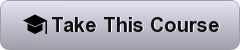Home Data Science Data Science: Probability

# Data Science: Probability

835
0Gain important foundational knowledge in probability theory, essential for data scientist, as you learn key concepts through a motivating case study on the financial crisis of 2007-08.

Harvard Online Course Highlights
• weeks long
• 2-4 hours per week
• Learn for FREE, Ugpradable
• Self-Paced
• Taught by: Rafael Irizarry, Professor of Biostatistics
• View Course Syllabus## Online Course Details:

In this course, part of our Professional Certificate Program in Data Science, you will learn valuable concepts in probability theory. The motivation for this course is the circumstances surrounding the financial crisis of 2007–2008. Part of what caused this financial crisis was that the risk of some securities sold by financial institutions was underestimated. To begin to understand this very complicated event, we need to understand the basics of probability.

We will introduce important concepts such as random variables, independence, Monte Carlo simulations, expected values, standard errors, and the Central Limit Theorem. These statistical concepts are fundamental to conducting statistical tests on data and understanding whether the data you are analyzing is likely occurring due to an experimental method or to chance.

Probability theory is the mathematical foundation of statistical inference which is indispensable for analyzing data affected by chance, and thus essential for data scientists.

### What you’ll learn

• Important concepts in probability theory including random variables and independence
• How to perform a Monte Carlo simulation
• The meaning of expected values and standard errors and how to compute them in R
• The importance of the Central Limit Theorem